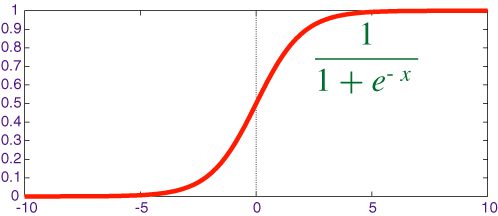# Logistic Regression（逻辑回归）原理及公式推导

Logistic Regression（逻辑回归）是机器学习中一个非常非常常见的模型，在实际生产环境中也常常被使用，是一种经典的分类模型（不是回归模型）。本文主要介绍了Logistic Regression（逻辑回归）模型的原理以及参数估计、公式推导方法。

## 模型构建

z=θ0+θ1x1+θ2x2+θ3x3...+θnxn=θTx

hθ(x)=11+ez=11+eθTx

y=11+ex

sigmoid的函数图形如下：hθ(x)$h_{\theta}(x)$<0.5 则说明当前数据属于A类；
hθ(x)$h_{\theta}(x)$>0.5 则说明当前数据属于B类。

P(y=1|x;θ)=hθ(x)

P(y=0|x;θ)=1hθ(x)

## 极大似然估计

P(y|x;θ)=(hθ(x))y(1hθ(x))1y

L(θ)=i=1mP(y(i)|x(i);θ)

L(θ)=i=1m(hθ(x(i)))y(i)(1hθ(x(i)))1y(i)

l(θ)=log(L(θ))=i=1mlog((hθ(x(i)))y(i))+log((1hθ(x(i)))1y(i))

l(θ)=log(L(θ))=i=1my(i)log(hθ(x(i)))+(1y(i))log(1hθ(x(i)))

J(θ)=1ml(θ)